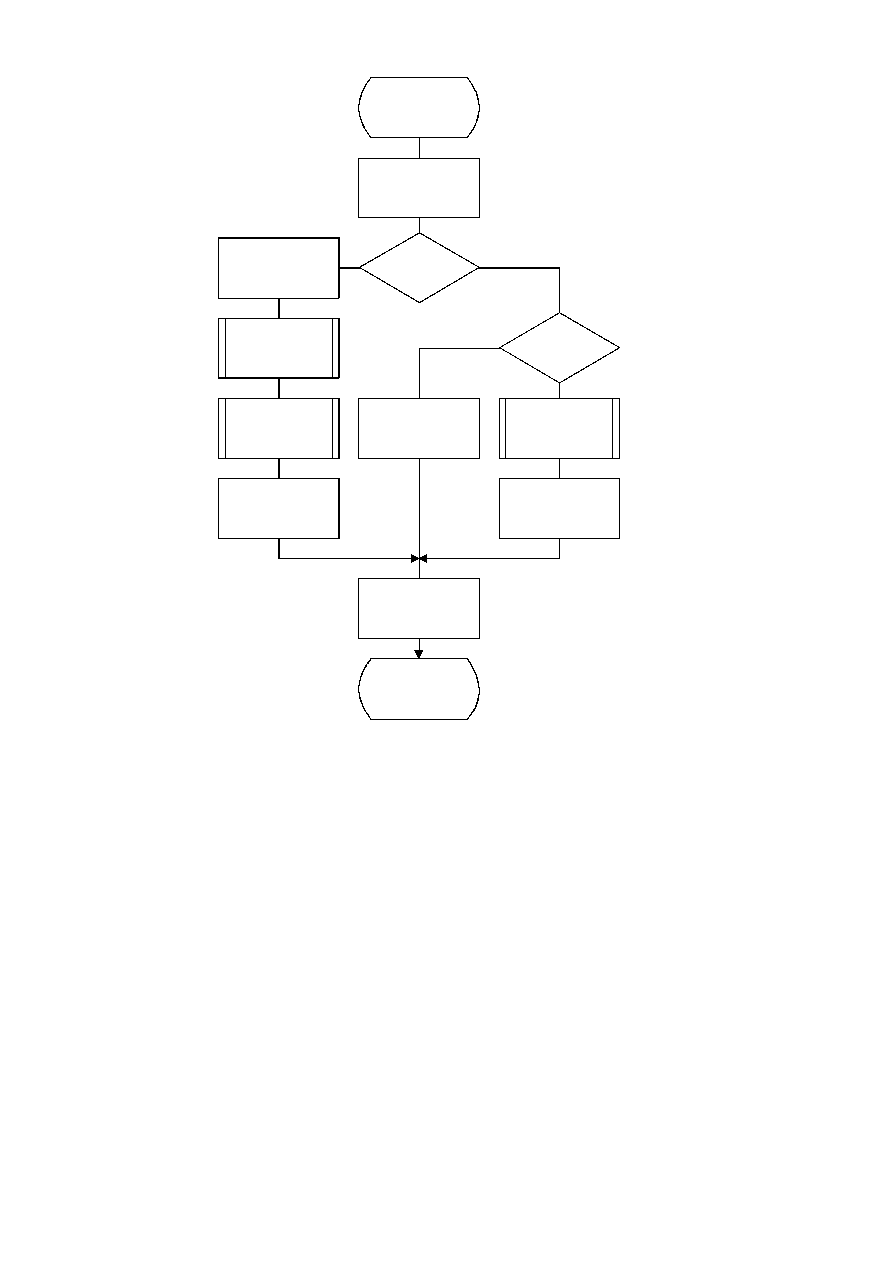ISO/IEC 10918-1 : 1993(E)
TISO1090-93/d046
Byte_out
T = SRL C 19
Yes
No
B = B + 1
Stuff_0
ST = ST + 1
Yes
No
Output_stacked_
zeros
BP = BP + 1
B = T
BP = BP + 1
B = T
C = C AND X'7FFFF'
Done
Output_stacked_
X'FF's
T > X'FF'
?
T = X'FF'
?
Figure D.8 ­ Byte_out procedure for encoder
Figure D.8 [D46], = 18 cm = 704.%
When the stack count reaches an upper bound determined by output channel capacity, the stack is emptied and the stacked
X'FF' bytes (and stuffed zero bytes) are added to the compressed data before the carry-over is resolved. If a carry-over
then occurs, the carry is added to the final stuffed zero, thereby converting the final X'FF00' sequence to the X'FF01'
temporary private marker. The entropy-coded segment must then be post-processed to resolve the carry-over and remove
the temporary marker code. For any reasonable bound on ST this post processing is very unlikely.
Referring to Figure D.8, the shift of the code register by 19 bits aligns the output bits with the low order bits of T. The
first test then determines if a carry-over has occurred. If so, the carry must be added to the previous output byte before
advancing the segment pointer BP. The Stuff_0 procedure stuffs a zero byte whenever the addition of the carry to the data
already in the entropy-coded segments creates a X'FF' byte. Any stacked output bytes ­ converted to zeros by the carry-
over ­ are then placed in the entropy-coded segment. Note that when the output byte is later transferred from T to the
entropy-coded segment (to byte B), the carry bit is ignored if it is set.
64
CCITT Rec. T.81 (1992 E)